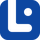# Articles related to "either"

### Either vs. Whether

This is one of the easiest grammatical points in the English language. But in some ways, it can be a little tricky. Let's start.

### Either vs. Both

The difference between 'either' and 'both' is actually very easy to understand. Follow the article to fully grasp the differences and similarities.

### Distributive Pronouns

Distributive pronouns refer to nouns separately rather than collectively in a group. In this lesson, we will learn all about them.

### Either vs. Too

You might have seen these two commonly-used words a lot. Each of them has its uses, similarities, and differences.

### Quantifiers

Quantifiers or Quantitatives are a type of determiners that are used with nouns. In this lesson, we will discover their uses, rules, and more in detail.

### Either

'Either' has many functions in the English grammar such as being a determiner. In this lesson, we will discover all about this commonly used word.

### Either vs. Neither

Both of them can act as determiners, pronouns, adverbs, or conjunctions. In this lesson, we will learn their similarities and differences.

### Either vs. Ither

It is rare to see one of these words in the standard English context. But, since it is a question on your mind, let's know more about them.

### Distributives

Distributives are determiners that indicate divided groups of people. In this lesson, we will learn uses, grammatical rules, and more.

### Pro-forms

Understanding pronouns enable us to understand pro-forms in English very well. Pro-forms are alternatives that are put in the position of words, phrases, etc.

### Correlative Conjunctions

Correlative conjunctions are pairs of words that join or correlate phrases or words with equal importance in a sentence.

### Each vs. Either

Actually, either and each are misused a lot. Let us get to know them and clarify their differences.